# LC Lowpass filter

#### xxxyyyba

Joined Aug 7, 2012
289
Hi!
Lowpass filter circuit is given: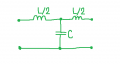Input signal to this circuit is: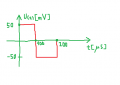L=36.4mH, C=85.4nF.
Calculate and draw output signal.

My idea is to:
1) Represent input signal in term of Fourier series (complex form),
2) Calculate transfer function for given circuit (for n-th harmonic),
Expression for output signal would be product of Fn of input signal (complex fourier coefficient) and transfer function of circuit.

1)
Here is input signal in term of Fourier series (complex form):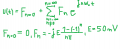2)
Here is transfer function for n-th harmonic:
(L'=L/2)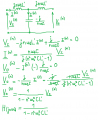Output signal is now: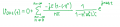First question: what is purpose of second inductor? We don't take into account when calculating transfer function.
Second question: If I plot output voltage I calculated, it doesn't match with voltage I got in simulation software. My calculations are related to stady state response of circuit, but it looks like it never goes to steady stade (I would say).
What to do in situations like this? Should I use some other method to find transient response (maybe Laplace transform?)?

Last edited:

#### RBR1317

Joined Nov 13, 2010
712
Purpose of the second inductor? Perhaps it is a graphic example for next time to not forget the termination impedance.

Analysis by Fourier series? Is the input waveform repeated continuously from -∞ to +∞ or is it a one-shot as shown? If the latter, then how are the Fourier coefficients turned on and off at the appropriate times? A time-limited non-repeating signal can be decomposed by computing the Fourier Integral, but that will produce a band spectra rather than the line spectra of the Fourier series. Band spectra won't find the time domain response.

So how good are you at solving second-order differential equations? Perhaps that is the purpose of the second inductor - to make it look complicated without needing a third-order differential equation. Note for the future: this is fairly simple to solve in the complex frequency domain, with an inverse Laplace transform to obtain the time-domain response.